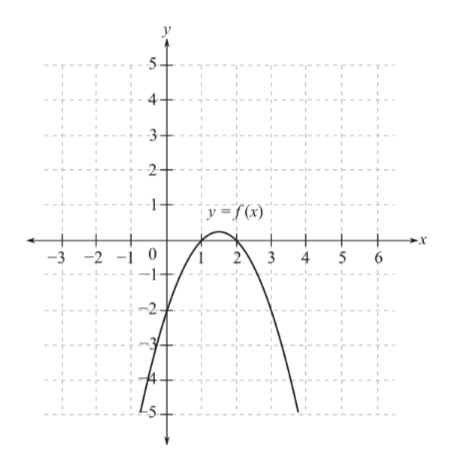### October 21st 2021 archive

In my precalc 11 this week, we learned about the quadratic formula. This is another way to solve quadratic equations, other than factoring or completing the square. The quadratic formula looks like this:It is easy to solve an equation using the quadratic formula because all you do is put in the numbers, and use algebra to find the variable. To do this, you must find out what numbers in the polynomial are A, B and C.  A is always the number with the x², B is always with the x, and C is always the last number, without a variable. Remember that when there is no number with an x, there is an invisible 1. Here are some examples of what A, B, and C are in the following polynomials.This is how to solve a polynomial using the quadratic formula:In the next equation, the quadratic formula is used, but the answers are 2 rational roots. This shows that the equation could have been factored from the start, rather than doing the quadratic formula, which sometimes takes longer. When you need to solve a quadratic equation, first check if the numbers can be factored, or if you can use the technique of completing the square. If not, using the quadratic formula will guarantee the correct answer.In my precalc 11 class this week, I learned about graphing, and how it can relate to a quadratic equation or function. A quadratic function is in the form y=ax² + bx + c

These are all quadratic equations: Even if they don’t look like it at the start, they can be moved around into the correct form.These graphs in curved shapes are called parabolas:When an equation creates a graph in a parabola, it is a quadratic function. Parabolas have a vertex, which is either the highest or lowest point. If it is the highest point on the graph, it is called the maximum point. If it is at the bottom, it is the minimum point. Here are some examples: